### Metals and Non-metals - Solutions

CBSE Class 10 Science

NCERT Solutions
Chapter - 3
Metals and Non-Metals

Page No. 40

1. Give an example of metal which

(i)   is a liquid at room temperature.

(ii)  can be easily cut with knife.

(iii) is best conductor of heat.

(iv) is poor conductor of heat.

Ans. (i) Mercury

(ii) Sodium

(iii) Silver

2. Explain the meaning of malleable and ductile.

Ans. A substance that can be beaten into thin sheets is said to be malleable. For example, iron, copper etc.

A substance that can be drawn into wires is called ductile. For example, gold, silver etc.

Page No. 46

1. Why is sodium kept immersed in kerosene oil?

Ans. Sodium reacts so vigorously with oxygen that it catches fire when kept in the opens. Hence, to protect accidental fires, it is kept immersed in kerosene oil.

2. Write equation for the reaction of

(i) Iron with steam

(ii) Calcium and potassium with water

Ans.

(i) 3Fe + 4H2$\to$ Fe3O+ 4H2

(ii) Ca + 2H2$\to$ Ca(OH)2 + H2

2K + 2H2$\to$ 2KOH + H2

3. Samples of four metals A, B, C and D were taken and added to the following solution one by one. The results obtained have been tabulated as follows: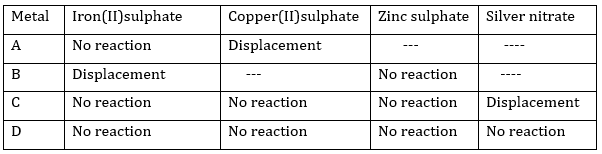Use the table given above to answer the f0llowing questions about metals A,B,C and D.

(i) Which is the most reactive metal?

(ii) What would you observe if B is added to a solution of Copper(II) sulphate?

(iii) Arrange the metals A, B,C and D in order of decreasing reactivity.

Ans. (i) B is the most reactive metal

(ii)If B is added to a solution of copper (II) sulphate, displacement reaction will take place. Blue colour of copper sulphate will fade and red –brown copper will settle down.

(iii) The decreasing order of reactivity is:

B> A> C >D

4. Which gas is produced when dilute hydrochloric acid is added to reactive metal?

Ans. Hydrogen gas is produced when dilute hydrochloric acid is added to a reactive metal.

5. What would you observe when zinc is added to a solution of iron(II) sulphate? Write the chemical reaction that takes place.

Ans. As zinc is more reactive than iron, displacement reaction will take place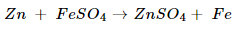Page No. 49

1. (i) Write the electro-dot structures for sodium, oxygen, and magnesium.

(ii) Show the formation of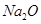and MgO by the transfer of electrons.

(iii) What are the ions present in these compounds?

Ans. (i) Electron-dot structure for sodium, oxygen and magnesium are

(ii)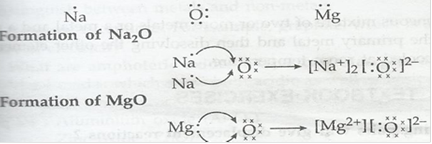(iii) Ions present in Na2O are Na+ and O2-

Ions present in MgO are Mg2+ and O2-

2. Why do ionic compounds have high melting points?

Ans. There are strong forces of attraction between oppositely charged ions in ionic compounds. Considerable amount of energy is required to break strong inter-ionic force of attraction. Therefore, they have high melting points.

Page No. 53

1. Define the following terms:

(i) Minerals

(ii) Ores and

(iii) Gangue

Ans. (i) Minerals- the element or compounds which occur naturally in the earth crest are known as minerals.

(ii) Ores- Minerals from which metal can be extracted profitably and easily are called ores.

(iii) Gangue- Impurities such as soil and sand which are present in the minerals are called gangue.

2. Name two metals which are found in nature in the free state.

Ans. Gold and Platinum

3. What chemical process is used for obtaining a metal from its oxide?

Ans. A metal is obtained from its oxide by the process of reduction.

Page No. 55

1. Metallic oxide of zinc, magnesium and copper were heated with following metals: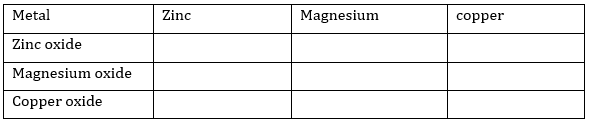In which case will you find displacement reactions taking place?

Ans. Based on the activity series of metals, the displacement reactions will take place as below: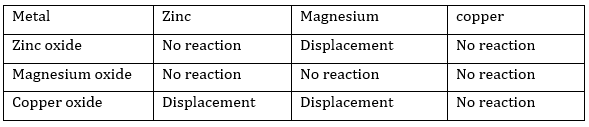2. Which metals do not corrode easily?

Ans. Metals which are placed at the bottom of activity series like silver, gold, platinum do not corrode easily.

3. What are alloys?

Ans. An alloy is homogenous mixture of two or more metals or metal and non-metal. It is obtained by first melting primary metal and then dissolving the other element in it in definite proportion.